NameFlagsCard.TypeDescription & Constraints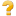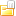Endpoint 0..*EndpointThe technical details of an endpoint that can be used for electronic services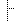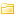meta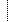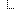lastUpdated 1..1instantWhen the resource version last changed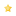extension 0..*ExtensionExtension
Slice: Unordered, Open by value:url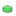endpoint-usecase S0..*(Complex)Endpoint Usecase
URL: http://hl7.org/fhir/us/davinci-pdex-plan-net/StructureDefinition/endpoint-usecasestatus S1..1codeactive | suspended | error | off | entered-in-error | test
Fixed Value: activeconnectionType S1..1CodingProtocol/Profile/Standard to be used with this endpoint connection
Binding: Endpoint Connection Types (extensible)name S0..1stringA name that this endpoint can be identified by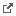managingOrganization S0..1Reference(Plan-Net Organization)Organization that manages this endpoint (might not be the organization that exposes the endpoint)contact S0..*ContactPointContact details for source (e.g. troubleshooting)system S0..1codephone | fax | email | pager | url | sms | othervalue S0..1stringThe actual contact point detailspayloadType S1..1CodeableConceptThe type of content that may be used at this endpoint (e.g. XDS Discharge summaries)
Binding: Endpoint Payload Types (extensible)payloadMimeType S0..*codeMimetype to send. If not specified, the content could be anything (including no payload, if the connectionType defined this)address S1..1urlThe technical base address for connecting to this endpointDocumentation for this format
<status value="active"/> <date value="2020-12-20T02:56:54+00:00"/> <publisher value="HL7 Financial Management Working Group"/> <contact> <name value="HL7 Financial Management Working Group"/> <telecom> <system value="url"/> <value value="http://www.hl7.org/Special/committees/fm/index.cfm"/> </telecom> <telecom> <system value="email"/> <value value="fm@lists.HL7.org"/> </telecom> </contact> <description value="The technical details of an endpoint that can be used for electronic services, such as a portal or FHIR REST services, messaging or operations, or DIRECT messaging."/> <jurisdiction> <coding> <system value="urn:iso:std:iso:3166"/> <code value="US"/> </coding> </jurisdiction> <fhirVersion value="4.0.1"/> <mapping> <identity value="rim"/> <uri value="http://hl7.org/v3"/> <name value="RIM Mapping"/> </mapping> <mapping> <identity value="w5"/> <uri value="http://hl7.org/fhir/fivews"/> <name value="FiveWs Pattern Mapping"/> </mapping> <kind value="resource"/> <abstract value="false"/> <type value="Endpoint"/> <baseDefinition value="http://hl7.org/fhir/StructureDefinition/Endpoint"/> <derivation value="constraint"/> <snapshot> <element id="Endpoint"> <path value="Endpoint"/> <short value="The technical details of an endpoint that can be used for electronic services"/> <definition value="The technical details of an endpoint that can be used for electronic services, such as for web services providing XDS.b or a REST endpoint for another FHIR server. This may include any security context information."/> <min value="0"/> <max value="*"/> <base> <path value="Endpoint"/> <min value="0"/> <max value="*"/> </base> <constraint> <key value="dom-2"/> <severity value="error"/> <human value="If the resource is contained in another resource, it SHALL NOT contain nested Resources"/> <expression value="contained.contained.empty()"/> <xpath value="not(parent::f:contained and f:contained)"/> <source value="http://hl7.org/fhir/StructureDefinition/DomainResource"/> </constraint> <constraint> <key value="dom-3"/> <severity value="error"/> <human value="If the resource is contained in another resource, it SHALL be referred to from elsewhere in the resource or SHALL refer to the containing resource"/> <expression value="contained.where((('#'+id in (%resource.descendants().reference | %resource.descendants().as(canonical) | %resource.descendants().as(uri) | %resource.descendants().as(url))) or descendants().where(reference = '#').exists() or descendants().where(as(canonical) = '#').exists() or descendants().where(as(canonical) = '#').exists()).not()).trace('unmatched', id).empty()"/> <xpath value="not(exists(for \$id in f:contained/*/f:id/@value return \$contained[not(parent::*/descendant::f:reference/@value=concat('#', \$contained/*/id/@value) or descendant::f:reference[@value='#'])]))"/> <source value="http://hl7.org/fhir/StructureDefinition/DomainResource"/> </constraint> <constraint> <key value="dom-4"/> <severity value="error"/> <human value="If a resource is contained in another resource, it SHALL NOT have a meta.versionId or a meta.lastUpdated"/> <expression value="contained.meta.versionId.empty() and contained.meta.lastUpdated.empty()"/> <xpath value="not(exists(f:contained/*/f:meta/f:versionId)) and not(exists(f:contained/*/f:meta/f:lastUpdated))"/> <source value="http://hl7.org/fhir/StructureDefinition/DomainResource"/> </constraint> <constraint> <key value="dom-5"/> <severity value="error"/> <human value="If a resource is contained in another resource, it SHALL NOT have a security label"/> <expression value="contained.meta.security.empty()"/> <xpath value="not(exists(f:contained/*/f:meta/f:security))"/> <source value="http://hl7.org/fhir/StructureDefinition/DomainResource"/> </constraint> <constraint> <extension url="http://hl7.org/fhir/StructureDefinition/elementdefinition-bestpractice"> <valueBoolean value="true"/> </extension> <extension url="http://hl7.org/fhir/StructureDefinition/elementdefinition-bestpractice-explanation"> <valueMarkdown value="When a resource has no narrative, only systems that fully understand the data can display the resource to a human safely. Including a human readable representation in the resource makes for a much more robust eco-system and cheaper handling of resources by intermediary systems. Some ecosystems restrict distribution of resources to only those systems that do fully understand the resources, and as a consequence implementers may believe that the narrative is superfluous. However experience shows that such eco-systems often open up to new participants over time."/> </extension> <key value="dom-6"/> <severity value="warning"/> <human value="A resource should have narrative for robust management"/> <expression value="text.`div`.exists()"/> <xpath value="exists(f:text/h:div)"/> <source value="http://hl7.org/fhir/StructureDefinition/DomainResource"/> </constraint> <isModifier value="false"/> <isSummary value="false"/> <mapping> <identity value="rim"/> <map value="Entity. Role, or Act"/> </mapping> <mapping> <identity value="rim"/> <map value="n/a"/> </mapping> </element> <element id="Endpoint.id"> <path value="Endpoint.id"/> <short value="Logical id of this artifact"/> <definition value="The logical id of the resource, as used in the URL for the resource. Once assigned, this value never changes."/> <comment value="The only time that a resource does not have an id is when it is being submitted to the server using a create operation."/> <min value="0"/> <max value="1"/> <base> <path value="Resource.id"/> <min value="0"/> <max value="1"/> </base> <type> <extension url="http://hl7.org/fhir/StructureDefinition/structuredefinition-fhir-type"> <valueUrl value="string"/> </extension> <code value="http://hl7.org/fhirpath/System.String"/> </type> <isModifier value="false"/> <isSummary value="true"/> </element> <element id="Endpoint.meta"> <path value="Endpoint.meta"/> <short value="Metadata about the resource"/> <definition value="The metadata about the resource. This is content that is maintained by the infrastructure. Changes to the content might not always be associated with version changes to the resource."/> <min value="0"/> <max value="1"/> <base> <path value="Resource.meta"/> <min value="0"/> <max value="1"/> </base> <type> <code value="Meta"/> </type> <constraint> <key value="ele-1"/> <severity value="error"/> <human value="All FHIR elements must have a @value or children"/> <expression value="hasValue() or (children().count() > id.count())"/> <xpath value="@value|f:*|h:div"/> <source value="http://hl7.org/fhir/StructureDefinition/Element"/> </constraint> <isModifier value="false"/> <isSummary value="true"/> </element> <element id="Endpoint.meta.id"> <path value="Endpoint.meta.id"/> <representation value="xmlAttr"/> <short value="Unique id for inter-element referencing"/> <definition value="Unique id for the element within a resource (for internal references). This may be any string value that does not contain spaces."/> <min value="0"/> <max value="1"/> <base> <path value="Element.id"/> <min value="0"/> <max value="1"/> </base> <type> <extension url="http://hl7.org/fhir/StructureDefinition/structuredefinition-fhir-type"> <valueUrl value="string"/> </extension> <code value="http://hl7.org/fhirpath/System.String"/> </type> <isModifier value="false"/> <isSummary value="false"/> <mapping> <identity value="rim"/> <map value="n/a"/> </mapping> </element> <element id="Endpoint.meta.extension"> <path value="Endpoint.meta.extension"/> <slicing> <discriminator> <type value="value"/> <path value="url"/> </discriminator> <description value="Extensions are always sliced by (at least) url"/> <rules value="open"/> </slicing> <short value="Additional content defined by implementations"/> <definition value="May be used to represent additional information that is not part of the basic definition of the element. To make the use of extensions safe and manageable, there is a strict set of governance applied to the definition and use of extensions. Though any implementer can define an extension, there is a set of requirements that SHALL be met as part of the definition of the extension."/> <comment value="There can be no stigma associated with the use of extensions by any application, project, or standard - regardless of the institution or jurisdiction that uses or defines the extensions. The use of extensions is what allows the FHIR specification to retain a core level of simplicity for everyone."/> <alias value="extensions"/> <alias value="user content"/> <min value="0"/> <max value="*"/> <base> <path value="Element.extension"/> <min value="0"/> <max value="*"/> </base> <type> <code value="Extension"/> </type> <constraint> <key value="ele-1"/> <severity value="error"/> <human value="All FHIR elements must have a @value or children"/> <expression value="hasValue() or (children().count() > id.count())"/> <xpath value="@value|f:*|h:div"/> <source value="http://hl7.org/fhir/StructureDefinition/Element"/> </constraint> <constraint> <key value="ext-1"/> <severity value="error"/> <human value="Must have either extensions or value[x], not both"/> <expression value="extension.exists() != value.exists()"/> <xpath value="exists(f:extension)!=exists(f:*[starts-with(local-name(.), "value")])"/> <source value="http://hl7.org/fhir/StructureDefinition/Extension"/> </constraint> <isModifier value="false"/> <isSummary value="false"/> <mapping> <identity value="rim"/> <map value="n/a"/> </mapping> </element> <element id="Endpoint.meta.versionId"> <path value="Endpoint.meta.versionId"/> <short value="Version specific identifier"/> <definition value="The version specific identifier, as it appears in the version portion of the URL. This value changes when the resource is created, updated, or deleted."/> <comment value="The server assigns this value, and ignores what the client specifies, except in the case that the server is imposing version integrity on updates/deletes."/> <min value="0"/> <max value="1"/> <base> <path value="Meta.versionId"/> <min value="0"/> <max value="1"/> </base> <type> <code value="id"/> </type> <constraint> <key value="ele-1"/> <severity value="error"/> <human value="All FHIR elements must have a @value or children"/> <expression value="hasValue() or (children().count() > id.count())"/> <xpath value="@value|f:*|h:div"/> <source value="http://hl7.org/fhir/StructureDefinition/Element"/> </constraint> <isModifier value="false"/> <isSummary value="true"/> </element> <element id="Endpoint.meta.lastUpdated"> <path value="Endpoint.meta.lastUpdated"/> <short value="When the resource version last changed"/> <definition value="When the resource last changed - e.g. when the version changed."/> <comment value="This value is always populated except when the resource is first being created. The server / resource manager sets this value; what a client provides is irrelevant. This is equivalent to the HTTP Last-Modified and SHOULD have the same value on a [read](http://hl7.org/fhir/R4/http.html#read) interaction."/> <min value="1"/> <max value="1"/> <base> <path value="Meta.lastUpdated"/> <min value="0"/> <max value="1"/> </base> <type> <code value="instant"/> </type> <constraint> <key value="ele-1"/> <severity value="error"/> <human value="All FHIR elements must have a @value or children"/> <expression value="hasValue() or (children().count() > id.count())"/> <xpath value="@value|f:*|h:div"/> <source value="http://hl7.org/fhir/StructureDefinition/Element"/> </constraint> <isModifier value="false"/> <isSummary value="true"/> </element> <element id="Endpoint.meta.source"> <path value="Endpoint.meta.source"/> <short value="Identifies where the resource comes from"/> <definition value="A uri that identifies the source system of the resource. This provides a minimal amount of [Provenance](http://hl7.org/fhir/R4/provenance.html#) information that can be used to track or differentiate the source of information in the resource. The source may identify another FHIR server, document, message, database, etc."/> <comment value="In the provenance resource, this corresponds to Provenance.entity.what[x]. The exact use of the source (and the implied Provenance.entity.role) is left to implementer discretion. Only one nominated source is allowed; for additional provenance details, a full Provenance resource should be used. This element can be used to indicate where the current master source of a resource that has a canonical URL if the resource is no longer hosted at the canonical URL."/> <min value="0"/> <max value="1"/> <base> <path value="Meta.source"/> <min value="0"/> <max value="1"/> </base> <type> <code value="uri"/> </type> <constraint> <key value="ele-1"/> <severity value="error"/> <human value="All FHIR elements must have a @value or children"/> <expression value="hasValue() or (children().count() > id.count())"/> <xpath value="@value|f:*|h:div"/> <source value="http://hl7.org/fhir/StructureDefinition/Element"/> </constraint> <isModifier value="false"/> <isSummary value="true"/> </element> <element id="Endpoint.meta.profile"> <path value="Endpoint.meta.profile"/> <short value="Profiles this resource claims to conform to"/> <definition value="A list of profiles (references to [StructureDefinition](http://hl7.org/fhir/R4/structuredefinition.html#) resources) that this resource claims to conform to. The URL is a reference to [StructureDefinition.url](http://hl7.org/fhir/R4/structuredefinition-definitions.html#StructureDefinition.url)."/> <comment value="It is up to the server and/or other infrastructure of policy to determine whether/how these claims are verified and/or updated over time. The list of profile URLs is a set."/> <min value="0"/> <max value="*"/> <base> <path value="Meta.profile"/> <min value="0"/> <max value="*"/> </base> <type> <code value="canonical"/> <targetProfile value="http://hl7.org/fhir/StructureDefinition/StructureDefinition"/> </type> <constraint> <key value="ele-1"/> <severity value="error"/> <human value="All FHIR elements must have a @value or children"/> <expression value="hasValue() or (children().count() > id.count())"/> <xpath value="@value|f:*|h:div"/> <source value="http://hl7.org/fhir/StructureDefinition/Element"/> </constraint> <isModifier value="false"/> <isSummary value="true"/> </element> <element id="Endpoint.meta.security"> <path value="Endpoint.meta.security"/> <short value="Security Labels applied to this resource"/> <definition value="Security labels applied to this resource. These tags connect specific resources to the overall security policy and infrastructure."/> <comment value="The security labels can be updated without changing the stated version of the resource. The list of security labels is a set. Uniqueness is based the system/code, and version and display are ignored."/> <min value="0"/> <max value="*"/> <base> <path value="Meta.security"/> <min value="0"/> <max value="*"/> </base> <type> <code value="Coding"/> </type> <constraint> <key value="ele-1"/> <severity value="error"/> <human value="All FHIR elements must have a @value or children"/> <expression value="hasValue() or (children().count() > id.count())"/> <xpath value="@value|f:*|h:div"/> <source value="http://hl7.org/fhir/StructureDefinition/Element"/> </constraint> <isModifier value="false"/> <isSummary value="true"/> <binding> <extension url="http://hl7.org/fhir/StructureDefinition/elementdefinition-bindingName"> <valueString value="SecurityLabels"/> </extension> <extension url="http://hl7.org/fhir/StructureDefinition/elementdefinition-isCommonBinding"> <valueBoolean value="true"/> </extension> <strength value="extensible"/> <description value="Security Labels from the Healthcare Privacy and Security Classification System."/> <valueSet value="http://hl7.org/fhir/ValueSet/security-labels"/> </binding> </element> <element id="Endpoint.meta.tag"> <path value="Endpoint.meta.tag"/> <short value="Tags applied to this resource"/> <definition value="Tags applied to this resource. Tags are intended to be used to identify and relate resources to process and workflow, and applications are not required to consider the tags when interpreting the meaning of a resource."/> <comment value="The tags can be updated without changing the stated version of the resource. The list of tags is a set. Uniqueness is based the system/code, and version and display are ignored."/> <min value="0"/> <max value="*"/> <base> <path value="Meta.tag"/> <min value="0"/> <max value="*"/> </base> <type> <code value="Coding"/> </type> <constraint> <key value="ele-1"/> <severity value="error"/> <human value="All FHIR elements must have a @value or children"/> <expression value="hasValue() or (children().count() > id.count())"/> <xpath value="@value|f:*|h:div"/> <source value="http://hl7.org/fhir/StructureDefinition/Element"/> </constraint> <isModifier value="false"/> <isSummary value="true"/> <binding> <extension url="http://hl7.org/fhir/StructureDefinition/elementdefinition-bindingName"> <valueString value="Tags"/> </extension> <strength value="example"/> <description value="Codes that represent various types of tags, commonly workflow-related; e.g. "Needs review by Dr. Jones"."/> <valueSet value="http://hl7.org/fhir/ValueSet/common-tags"/> </binding> </element> <element id="Endpoint.implicitRules"> <path value="Endpoint.implicitRules"/> <short value="A set of rules under which this content was created"/> <definition value="A reference to a set of rules that were followed when the resource was constructed, and which must be understood when processing the content. Often, this is a reference to an implementation guide that defines the special rules along with other profiles etc."/> <comment value="Asserting this rule set restricts the content to be only understood by a limited set of trading partners. This inherently limits the usefulness of the data in the long term. However, the existing health eco-system is highly fractured, and not yet ready to define, collect, and exchange data in a generally computable sense. Wherever possible, implementers and/or specification writers should avoid using this element. Often, when used, the URL is a reference to an implementation guide that defines these special rules as part of it's narrative along with other profiles, value sets, etc."/> <min value="0"/> <max value="1"/> <base> <path value="Resource.implicitRules"/> <min value="0"/> <max value="1"/> </base> <type> <code value="uri"/> </type> <constraint> <key value="ele-1"/> <severity value="error"/> <human value="All FHIR elements must have a @value or children"/> <expression value="hasValue() or (children().count() > id.count())"/> <xpath value="@value|f:*|h:div"/> <source value="http://hl7.org/fhir/StructureDefinition/Element"/> </constraint> <isModifier value="true"/> <isModifierReason value="This element is labeled as a modifier because the implicit rules may provide additional knowledge about the resource that modifies it's meaning or interpretation"/> <isSummary value="true"/> </element> <element id="Endpoint.language"> <path value="Endpoint.language"/> <short value="Language of the resource content"/> <definition value="The base language in which the resource is written."/> <comment value="Language is provided to support indexing and accessibility (typically, services such as text to speech use the language tag). The html language tag in the narrative applies to the narrative. The language tag on the resource may be used to specify the language of other presentations generated from the data in the resource. Not all the content has to be in the base language. The Resource.language should not be assumed to apply to the narrative automatically. If a language is specified, it should it also be specified on the div element in the html (see rules in HTML5 for information about the relationship between xml:lang and the html lang attribute)."/> <min value="0"/> <max value="1"/> <base> <path value="Resource.language"/> <min value="0"/> <max value="1"/> </base> <type> <code value="code"/> </type> <constraint> <key value="ele-1"/> <severity value="error"/> <human value="All FHIR elements must have a @value or children"/> <expression value="hasValue() or (children().count() > id.count())"/> <xpath value="@value|f:*|h:div"/> <source value="http://hl7.org/fhir/StructureDefinition/Element"/> </constraint> <isModifier value="false"/> <isSummary value="false"/> <binding> <extension url="http://hl7.org/fhir/StructureDefinition/elementdefinition-maxValueSet"> <valueCanonical value="http://hl7.org/fhir/ValueSet/all-languages"/> </extension> <extension url="http://hl7.org/fhir/StructureDefinition/elementdefinition-bindingName"> <valueString value="Language"/> </extension> <extension url="http://hl7.org/fhir/StructureDefinition/elementdefinition-isCommonBinding"> <valueBoolean value="true"/> </extension> <strength value="preferred"/> <description value="A human language."/> <valueSet value="http://hl7.org/fhir/ValueSet/languages"/> </binding> </element> <element id="Endpoint.text"> <path value="Endpoint.text"/> <short value="Text summary of the resource, for human interpretation"/> <definition value="A human-readable narrative that contains a summary of the resource and can be used to represent the content of the resource to a human. The narrative need not encode all the structured data, but is required to contain sufficient detail to make it "clinically safe" for a human to just read the narrative. Resource definitions may define what content should be represented in the narrative to ensure clinical safety."/> <comment value="Contained resources do not have narrative. Resources that are not contained SHOULD have a narrative. In some cases, a resource may only have text with little or no additional discrete data (as long as all minOccurs=1 elements are satisfied). This may be necessary for data from legacy systems where information is captured as a "text blob" or where text is additionally entered raw or narrated and encoded information is added later."/> <alias value="narrative"/> <alias value="html"/> <alias value="xhtml"/> <alias value="display"/> <min value="0"/> <max value="1"/> <base> <path value="DomainResource.text"/> <min value="0"/> <max value="1"/> </base> <type> <code value="Narrative"/> </type> <constraint> <key value="ele-1"/> <severity value="error"/> <human value="All FHIR elements must have a @value or children"/> <expression value="hasValue() or (children().count() > id.count())"/> <xpath value="@value|f:*|h:div"/> <source value="http://hl7.org/fhir/StructureDefinition/Element"/> </constraint> <isModifier value="false"/> <isSummary value="false"/> <mapping> <identity value="rim"/> <map value="Act.text?"/> </mapping> </element> <element id="Endpoint.contained"> <path value="Endpoint.contained"/> <short value="Contained, inline Resources"/> <definition value="These resources do not have an independent existence apart from the resource that contains them - they cannot be identified independently, and nor can they have their own independent transaction scope."/> <comment value="This should never be done when the content can be identified properly, as once identification is lost, it is extremely difficult (and context dependent) to restore it again. Contained resources may have profiles and tags In their meta elements, but SHALL NOT have security labels."/> <alias value="inline resources"/> <alias value="anonymous resources"/> <alias value="contained resources"/> <min value="0"/> <max value="*"/> <base> <path value="DomainResource.contained"/> <min value="0"/> <max value="*"/> </base> <type> <code value="Resource"/> </type> <isModifier value="false"/> <isSummary value="false"/> <mapping> <identity value="rim"/> <map value="N/A"/> </mapping> </element> <element id="Endpoint.extension"> <path value="Endpoint.extension"/> <slicing> <discriminator> <type value="value"/> <path value="url"/> </discriminator> <ordered value="false"/> <rules value="open"/> </slicing> <short value="Extension"/> <definition value="An Extension"/> <min value="0"/> <max value="*"/> <base> <path value="DomainResource.extension"/> <min value="0"/> <max value="*"/> </base> <type> <code value="Extension"/> </type> <constraint> <key value="ele-1"/> <severity value="error"/> <human value="All FHIR elements must have a @value or children"/> <expression value="hasValue() or (children().count() > id.count())"/> <xpath value="@value|f:*|h:div"/> <source value="http://hl7.org/fhir/StructureDefinition/Element"/> </constraint> <constraint> <key value="ext-1"/> <severity value="error"/> <human value="Must have either extensions or value[x], not both"/> <expression value="extension.exists() != value.exists()"/> <xpath value="exists(f:extension)!=exists(f:*[starts-with(local-name(.), "value")])"/> <source value="http://hl7.org/fhir/StructureDefinition/Extension"/> </constraint> <isModifier value="false"/> <isSummary value="false"/> </element> <element id="Endpoint.extension:endpoint-usecase"> <path value="Endpoint.extension"/> <sliceName value="endpoint-usecase"/> <short value="Endpoint Usecase"/> <definition value="Optional Extension Element - found in all resources."/> <min value="0"/> <max value="*"/> <base> <path value="DomainResource.extension"/> <min value="0"/> <max value="*"/> </base> <type> <code value="Extension"/> <profile value="http://hl7.org/fhir/us/davinci-pdex-plan-net/StructureDefinition/endpoint-usecase"/> </type> <condition value="ele-1"/> <constraint> <key value="ele-1"/> <severity value="error"/> <human value="All FHIR elements must have a @value or children"/> <expression value="hasValue() or (children().count() > id.count())"/> <xpath value="@value|f:*|h:div"/> <source value="http://hl7.org/fhir/StructureDefinition/Element"/> </constraint> <constraint> <key value="ext-1"/> <severity value="error"/> <human value="Must have either extensions or value[x], not both"/> <expression value="extension.exists() != value.exists()"/> <xpath value="exists(f:extension)!=exists(f:*[starts-with(local-name(.), 'value')])"/> <source value="http://hl7.org/fhir/StructureDefinition/Endpoint"/> </constraint> <mustSupport value="true"/> <isModifier value="false"/> <mapping> <identity value="rim"/> <map value="n/a"/> </mapping> <mapping> <identity value="rim"/> <map value="N/A"/> </mapping> </element> <element id="Endpoint.modifierExtension"> <path value="Endpoint.modifierExtension"/> <short value="Extensions that cannot be ignored"/> <definition value="May be used to represent additional information that is not part of the basic definition of the resource and that modifies the understanding of the element that contains it and/or the understanding of the containing element's descendants. Usually modifier elements provide negation or qualification. To make the use of extensions safe and manageable, there is a strict set of governance applied to the definition and use of extensions. Though any implementer is allowed to define an extension, there is a set of requirements that SHALL be met as part of the definition of the extension. Applications processing a resource are required to check for modifier extensions. Modifier extensions SHALL NOT change the meaning of any elements on Resource or DomainResource (including cannot change the meaning of modifierExtension itself)."/> <comment value="There can be no stigma associated with the use of extensions by any application, project, or standard - regardless of the institution or jurisdiction that uses or defines the extensions. The use of extensions is what allows the FHIR specification to retain a core level of simplicity for everyone."/> <requirements value="Modifier extensions allow for extensions that *cannot* be safely ignored to be clearly distinguished from the vast majority of extensions which can be safely ignored. This promotes interoperability by eliminating the need for implementers to prohibit the presence of extensions. For further information, see the [definition of modifier extensions](http://hl7.org/fhir/R4/extensibility.html#modifierExtension)."/> <alias value="extensions"/> <alias value="user content"/> <min value="0"/> <max value="*"/> <base> <path value="DomainResource.modifierExtension"/> <min value="0"/> <max value="*"/> </base> <type> <code value="Extension"/> </type> <constraint> <key value="ele-1"/> <severity value="error"/> <human value="All FHIR elements must have a @value or children"/> <expression value="hasValue() or (children().count() > id.count())"/> <xpath value="@value|f:*|h:div"/> <source value="http://hl7.org/fhir/StructureDefinition/Element"/> </constraint> <constraint> <key value="ext-1"/> <severity value="error"/> <human value="Must have either extensions or value[x], not both"/> <expression value="extension.exists() != value.exists()"/> <xpath value="exists(f:extension)!=exists(f:*[starts-with(local-name(.), "value")])"/> <source value="http://hl7.org/fhir/StructureDefinition/Extension"/> </constraint> <isModifier value="true"/> <isModifierReason value="Modifier extensions are expected to modify the meaning or interpretation of the resource that contains them"/> <isSummary value="false"/> <mapping> <identity value="rim"/> <map value="N/A"/> </mapping> </element> <element id="Endpoint.identifier"> <path value="Endpoint.identifier"/> <short value="Identifies this endpoint across multiple systems"/> <definition value="Identifier for the organization that is used to identify the endpoint across multiple disparate systems."/> <min value="0"/> <max value="*"/> <base> <path value="Endpoint.identifier"/> <min value="0"/> <max value="*"/> </base> <type> <code value="Identifier"/> </type> <constraint> <key value="ele-1"/> <severity value="error"/> <human value="All FHIR elements must have a @value or children"/> <expression value="hasValue() or (children().count() > id.count())"/> <xpath value="@value|f:*|h:div"/> <source value="http://hl7.org/fhir/StructureDefinition/Element"/> </constraint> <isModifier value="false"/> <isSummary value="true"/> <mapping> <identity value="w5"/> <map value="FiveWs.identifier"/> </mapping> <mapping> <identity value="rim"/> <map value="n/a"/> </mapping> </element> <element id="Endpoint.status"> <path value="Endpoint.status"/> <short value="active | suspended | error | off | entered-in-error | test"/> <definition value="active | suspended | error | off | test."/> <comment value="This element is labeled as a modifier because the status contains codes that mark the endpoint as not currently valid."/> <min value="1"/> <max value="1"/> <base> <path value="Endpoint.status"/> <min value="1"/> <max value="1"/> </base> <type> <code value="code"/> </type> <fixedCode value="active"/> <constraint> <key value="ele-1"/> <severity value="error"/> <human value="All FHIR elements must have a @value or children"/> <expression value="hasValue() or (children().count() > id.count())"/> <xpath value="@value|f:*|h:div"/> <source value="http://hl7.org/fhir/StructureDefinition/Element"/> </constraint> <mustSupport value="true"/> <isModifier value="true"/> <isModifierReason value="This element is labelled as a modifier because it is a status element that contains status entered-in-error which means that the resource should not be treated as valid"/> <isSummary value="true"/> <binding> <extension url="http://hl7.org/fhir/StructureDefinition/elementdefinition-bindingName"> <valueString value="EndpointStatus"/> </extension> <strength value="required"/> <description value="The status of the endpoint."/> <valueSet value="http://hl7.org/fhir/ValueSet/endpoint-status|4.0.1"/> </binding> <mapping> <identity value="w5"/> <map value="FiveWs.status"/> </mapping> <mapping> <identity value="rim"/> <map value="n/a"/> </mapping> </element> <element id="Endpoint.connectionType"> <path value="Endpoint.connectionType"/> <short value="Protocol/Profile/Standard to be used with this endpoint connection"/> <definition value="A coded value that represents the technical details of the usage of this endpoint, such as what WSDLs should be used in what way. (e.g. XDS.b/DICOM/cds-hook)."/> <comment value="For additional connectivity details for the protocol, extensions will be used at this point, as in the XDS example."/> <min value="1"/> <max value="1"/> <base> <path value="Endpoint.connectionType"/> <min value="1"/> <max value="1"/> </base> <type> <code value="Coding"/> </type> <constraint> <key value="ele-1"/> <severity value="error"/> <human value="All FHIR elements must have a @value or children"/> <expression value="hasValue() or (children().count() > id.count())"/> <xpath value="@value|f:*|h:div"/> <source value="http://hl7.org/fhir/StructureDefinition/Element"/> </constraint> <mustSupport value="true"/> <isModifier value="false"/> <isSummary value="true"/> <binding> <extension url="http://hl7.org/fhir/StructureDefinition/elementdefinition-minValueSet"> <valueCanonical value="http://hl7.org/fhir/us/davinci-pdex-plan-net/ValueSet/MinEndpointConnectionTypeVS"/> </extension> <strength value="extensible"/> <valueSet value="http://hl7.org/fhir/us/davinci-pdex-plan-net/ValueSet/EndpointConnectionTypeVS"/> </binding> <mapping> <identity value="w5"/> <map value="FiveWs.class"/> </mapping> <mapping> <identity value="rim"/> <map value="n/a"/> </mapping> </element> <element id="Endpoint.name"> <path value="Endpoint.name"/> <short value="A name that this endpoint can be identified by"/> <definition value="A friendly name that this endpoint can be referred to with."/> <min value="0"/> <max value="1"/> <base> <path value="Endpoint.name"/> <min value="0"/> <max value="1"/> </base> <type> <code value="string"/> </type> <constraint> <key value="ele-1"/> <severity value="error"/> <human value="All FHIR elements must have a @value or children"/> <expression value="hasValue() or (children().count() > id.count())"/> <xpath value="@value|f:*|h:div"/> <source value="http://hl7.org/fhir/StructureDefinition/Element"/> </constraint> <mustSupport value="true"/> <isModifier value="false"/> <isSummary value="true"/> <mapping> <identity value="w5"/> <map value="FiveWs.what[x]"/> </mapping> <mapping> <identity value="rim"/> <map value="n/a"/> </mapping> </element> <element id="Endpoint.managingOrganization"> <path value="Endpoint.managingOrganization"/> <short value="Organization that manages this endpoint (might not be the organization that exposes the endpoint)"/> <definition value="The organization that manages this endpoint (even if technically another organization is hosting this in the cloud, it is the organization associated with the data)."/> <comment value="This property is not typically used when searching for Endpoint resources for usage. The typical usage is via the reference from an applicable Organization/Location/Practitioner resource, which is where the context is provided. Multiple Locations may reference a single endpoint, and don't have to be within the same organization resource, but most likely within the same organizational hierarchy."/> <min value="0"/> <max value="1"/> <base> <path value="Endpoint.managingOrganization"/> <min value="0"/> <max value="1"/> </base> <type> <code value="Reference"/> <targetProfile value="http://hl7.org/fhir/us/davinci-pdex-plan-net/StructureDefinition/plannet-Organization"/> </type> <constraint> <key value="ele-1"/> <severity value="error"/> <human value="All FHIR elements must have a @value or children"/> <expression value="hasValue() or (children().count() > id.count())"/> <xpath value="@value|f:*|h:div"/> <source value="http://hl7.org/fhir/StructureDefinition/Element"/> </constraint> <mustSupport value="true"/> <isModifier value="false"/> <isSummary value="true"/> <mapping> <identity value="rim"/> <map value="n/a"/> </mapping> </element> <element id="Endpoint.contact"> <path value="Endpoint.contact"/> <short value="Contact details for source (e.g. troubleshooting)"/> <definition value="Contact details for a human to contact about the subscription. The primary use of this for system administrator troubleshooting."/> <min value="0"/> <max value="*"/> <base> <path value="Endpoint.contact"/> <min value="0"/> <max value="*"/> </base> <type> <code value="ContactPoint"/> </type> <constraint> <key value="ele-1"/> <severity value="error"/> <human value="All FHIR elements must have a @value or children"/> <expression value="hasValue() or (children().count() > id.count())"/> <xpath value="@value|f:*|h:div"/> <source value="http://hl7.org/fhir/StructureDefinition/Element"/> </constraint> <mustSupport value="true"/> <isModifier value="false"/> <isSummary value="false"/> <mapping> <identity value="rim"/> <map value="n/a"/> </mapping> </element> <element id="Endpoint.contact.id"> <path value="Endpoint.contact.id"/> <representation value="xmlAttr"/> <short value="Unique id for inter-element referencing"/> <definition value="Unique id for the element within a resource (for internal references). This may be any string value that does not contain spaces."/> <min value="0"/> <max value="1"/> <base> <path value="Element.id"/> <min value="0"/> <max value="1"/> </base> <type> <extension url="http://hl7.org/fhir/StructureDefinition/structuredefinition-fhir-type"> <valueUrl value="string"/> </extension> <code value="http://hl7.org/fhirpath/System.String"/> </type> <isModifier value="false"/> <isSummary value="false"/> <mapping> <identity value="rim"/> <map value="n/a"/> </mapping> </element> <element id="Endpoint.contact.extension"> <path value="Endpoint.contact.extension"/> <slicing> <discriminator> <type value="value"/> <path value="url"/> </discriminator> <description value="Extensions are always sliced by (at least) url"/> <rules value="open"/> </slicing> <short value="Additional content defined by implementations"/> <definition value="May be used to represent additional information that is not part of the basic definition of the element. To make the use of extensions safe and manageable, there is a strict set of governance applied to the definition and use of extensions. Though any implementer can define an extension, there is a set of requirements that SHALL be met as part of the definition of the extension."/> <comment value="There can be no stigma associated with the use of extensions by any application, project, or standard - regardless of the institution or jurisdiction that uses or defines the extensions. The use of extensions is what allows the FHIR specification to retain a core level of simplicity for everyone."/> <alias value="extensions"/> <alias value="user content"/> <min value="0"/> <max value="*"/> <base> <path value="Element.extension"/> <min value="0"/> <max value="*"/> </base> <type> <code value="Extension"/> </type> <constraint> <key value="ele-1"/> <severity value="error"/> <human value="All FHIR elements must have a @value or children"/> <expression value="hasValue() or (children().count() > id.count())"/> <xpath value="@value|f:*|h:div"/> <source value="http://hl7.org/fhir/StructureDefinition/Element"/> </constraint> <constraint> <key value="ext-1"/> <severity value="error"/> <human value="Must have either extensions or value[x], not both"/> <expression value="extension.exists() != value.exists()"/> <xpath value="exists(f:extension)!=exists(f:*[starts-with(local-name(.), "value")])"/> <source value="http://hl7.org/fhir/StructureDefinition/Extension"/> </constraint> <isModifier value="false"/> <isSummary value="false"/> <mapping> <identity value="rim"/> <map value="n/a"/> </mapping> </element> <element id="Endpoint.contact.system"> <path value="Endpoint.contact.system"/> <short value="phone | fax | email | pager | url | sms | other"/> <definition value="Telecommunications form for contact point - what communications system is required to make use of the contact."/> <min value="0"/> <max value="1"/> <base> <path value="ContactPoint.system"/> <min value="0"/> <max value="1"/> </base> <type> <code value="code"/> </type> <condition value="cpt-2"/> <constraint> <key value="ele-1"/> <severity value="error"/> <human value="All FHIR elements must have a @value or children"/> <expression value="hasValue() or (children().count() > id.count())"/> <xpath value="@value|f:*|h:div"/> <source value="http://hl7.org/fhir/StructureDefinition/Element"/> </constraint> <mustSupport value="true"/> <isModifier value="false"/> <isSummary value="true"/> <binding> <extension url="http://hl7.org/fhir/StructureDefinition/elementdefinition-bindingName"> <valueString value="ContactPointSystem"/> </extension> <strength value="required"/> <description value="Telecommunications form for contact point."/> <valueSet value="http://hl7.org/fhir/ValueSet/contact-point-system|4.0.1"/> </binding> <mapping> <identity value="v2"/> <map value="XTN.3"/> </mapping> <mapping> <identity value="rim"/> <map value="./scheme"/> </mapping> <mapping> <identity value="servd"/> <map value="./ContactPointType"/> </mapping> </element> <element id="Endpoint.contact.value"> <path value="Endpoint.contact.value"/> <short value="The actual contact point details"/> <definition value="The actual contact point details, in a form that is meaningful to the designated communication system (i.e. phone number or email address)."/> <comment value="Additional text data such as phone extension numbers, or notes about use of the contact are sometimes included in the value."/> <requirements value="Need to support legacy numbers that are not in a tightly controlled format."/> <min value="0"/> <max value="1"/> <base> <path value="ContactPoint.value"/> <min value="0"/> <max value="1"/> </base> <type> <code value="string"/> </type> <constraint> <key value="ele-1"/> <severity value="error"/> <human value="All FHIR elements must have a @value or children"/> <expression value="hasValue() or (children().count() > id.count())"/> <xpath value="@value|f:*|h:div"/> <source value="http://hl7.org/fhir/StructureDefinition/Element"/> </constraint> <mustSupport value="true"/> <isModifier value="false"/> <isSummary value="true"/> <mapping> <identity value="v2"/> <map value="XTN.1 (or XTN.12)"/> </mapping> <mapping> <identity value="rim"/> <map value="./url"/> </mapping> <mapping> <identity value="servd"/> <map value="./Value"/> </mapping> </element> <element id="Endpoint.contact.use"> <path value="Endpoint.contact.use"/> <short value="home | work | temp | old | mobile - purpose of this contact point"/> <definition value="Identifies the purpose for the contact point."/> <comment value="Applications can assume that a contact is current unless it explicitly says that it is temporary or old."/> <requirements value="Need to track the way a person uses this contact, so a user can choose which is appropriate for their purpose."/> <min value="0"/> <max value="1"/> <base> <path value="ContactPoint.use"/> <min value="0"/> <max value="1"/> </base> <type> <code value="code"/> </type> <constraint> <key value="ele-1"/> <severity value="error"/> <human value="All FHIR elements must have a @value or children"/> <expression value="hasValue() or (children().count() > id.count())"/> <xpath value="@value|f:*|h:div"/> <source value="http://hl7.org/fhir/StructureDefinition/Element"/> </constraint> <isModifier value="true"/> <isModifierReason value="This is labeled as "Is Modifier" because applications should not mistake a temporary or old contact etc.for a current/permanent one"/> <isSummary value="true"/> <binding> <extension url="http://hl7.org/fhir/StructureDefinition/elementdefinition-bindingName"> <valueString value="ContactPointUse"/> </extension> <strength value="required"/> <description value="Use of contact point."/> <valueSet value="http://hl7.org/fhir/ValueSet/contact-point-use|4.0.1"/> </binding> <mapping> <identity value="v2"/> <map value="XTN.2 - but often indicated by field"/> </mapping> <mapping> <identity value="rim"/> <map value="unique(./use)"/> </mapping> <mapping> <identity value="servd"/> <map value="./ContactPointPurpose"/> </mapping> </element> <element id="Endpoint.contact.rank"> <path value="Endpoint.contact.rank"/> <short value="Specify preferred order of use (1 = highest)"/> <definition value="Specifies a preferred order in which to use a set of contacts. ContactPoints with lower rank values are more preferred than those with higher rank values."/> <comment value="Note that rank does not necessarily follow the order in which the contacts are represented in the instance."/> <min value="0"/> <max value="1"/> <base> <path value="ContactPoint.rank"/> <min value="0"/> <max value="1"/> </base> <type> <code value="positiveInt"/> </type> <constraint> <key value="ele-1"/> <severity value="error"/> <human value="All FHIR elements must have a @value or children"/> <expression value="hasValue() or (children().count() > id.count())"/> <xpath value="@value|f:*|h:div"/> <source value="http://hl7.org/fhir/StructureDefinition/Element"/> </constraint> <isModifier value="false"/> <isSummary value="true"/> <mapping> <identity value="v2"/> <map value="n/a"/> </mapping> <mapping> <identity value="rim"/> <map value="n/a"/> </mapping> </element> <element id="Endpoint.contact.period"> <path value="Endpoint.contact.period"/> <short value="Time period when the contact point was/is in use"/> <definition value="Time period when the contact point was/is in use."/> <min value="0"/> <max value="1"/> <base> <path value="ContactPoint.period"/> <min value="0"/> <max value="1"/> </base> <type> <code value="Period"/> </type> <constraint> <key value="ele-1"/> <severity value="error"/> <human value="All FHIR elements must have a @value or children"/> <expression value="hasValue() or (children().count() > id.count())"/> <xpath value="@value|f:*|h:div"/> <source value="http://hl7.org/fhir/StructureDefinition/Element"/> </constraint> <isModifier value="false"/> <isSummary value="true"/> <mapping> <identity value="v2"/> <map value="N/A"/> </mapping> <mapping> <identity value="rim"/> <map value="./usablePeriod[type="IVL<TS>"]"/> </mapping> <mapping> <identity value="servd"/> <map value="./StartDate and ./EndDate"/> </mapping> </element> <element id="Endpoint.period"> <path value="Endpoint.period"/> <short value="Interval the endpoint is expected to be operational"/> <definition value="The interval during which the endpoint is expected to be operational."/> <min value="0"/> <max value="1"/> <base> <path value="Endpoint.period"/> <min value="0"/> <max value="1"/> </base> <type> <code value="Period"/> </type> <constraint> <key value="ele-1"/> <severity value="error"/> <human value="All FHIR elements must have a @value or children"/> <expression value="hasValue() or (children().count() > id.count())"/> <xpath value="@value|f:*|h:div"/> <source value="http://hl7.org/fhir/StructureDefinition/Element"/> </constraint> <isModifier value="false"/> <isSummary value="true"/> <mapping> <identity value="w5"/> <map value="FiveWs.done[x]"/> </mapping> <mapping> <identity value="rim"/> <map value="n/a"/> </mapping> </element> <element id="Endpoint.payloadType"> <path value="Endpoint.payloadType"/> <short value="The type of content that may be used at this endpoint (e.g. XDS Discharge summaries)"/> <definition value="The payload type describes the acceptable content that can be communicated on the endpoint."/> <comment value="The payloadFormat describes the serialization format of the data, where the payloadType indicates the specific document/schema that is being transferred; e.g. DischargeSummary or CarePlan."/> <min value="1"/> <max value="1"/> <base> <path value="Endpoint.payloadType"/> <min value="1"/> <max value="*"/> </base> <type> <code value="CodeableConcept"/> </type> <constraint> <key value="ele-1"/> <severity value="error"/> <human value="All FHIR elements must have a @value or children"/> <expression value="hasValue() or (children().count() > id.count())"/> <xpath value="@value|f:*|h:div"/> <source value="http://hl7.org/fhir/StructureDefinition/Element"/> </constraint> <mustSupport value="true"/> <isModifier value="false"/> <isSummary value="true"/> <binding> <strength value="extensible"/> <valueSet value="http://hl7.org/fhir/us/davinci-pdex-plan-net/ValueSet/EndpointPayloadTypeVS"/> </binding> <mapping> <identity value="rim"/> <map value="n/a"/> </mapping> </element> <element id="Endpoint.payloadMimeType"> <path value="Endpoint.payloadMimeType"/> <short value="Mimetype to send. If not specified, the content could be anything (including no payload, if the connectionType defined this)"/> <definition value="The mime type to send the payload in - e.g. application/fhir+xml, application/fhir+json. If the mime type is not specified, then the sender could send any content (including no content depending on the connectionType)."/> <comment value="Sending the payload has obvious security consequences. The server is responsible for ensuring that the content is appropriately secured."/> <min value="0"/> <max value="*"/> <base> <path value="Endpoint.payloadMimeType"/> <min value="0"/> <max value="*"/> </base> <type> <code value="code"/> </type> <constraint> <key value="ele-1"/> <severity value="error"/> <human value="All FHIR elements must have a @value or children"/> <expression value="hasValue() or (children().count() > id.count())"/> <xpath value="@value|f:*|h:div"/> <source value="http://hl7.org/fhir/StructureDefinition/Element"/> </constraint> <mustSupport value="true"/> <isModifier value="false"/> <isSummary value="true"/> <binding> <extension url="http://hl7.org/fhir/StructureDefinition/elementdefinition-bindingName"> <valueString value="MimeType"/> </extension> <extension url="http://hl7.org/fhir/StructureDefinition/elementdefinition-isCommonBinding"> <valueBoolean value="true"/> </extension> <strength value="required"/> <description value="The mime type of an attachment. Any valid mime type is allowed."/> <valueSet value="http://hl7.org/fhir/ValueSet/mimetypes|4.0.1"/> </binding> <mapping> <identity value="rim"/> <map value="n/a"/> </mapping> </element> <element id="Endpoint.address"> <path value="Endpoint.address"/> <short value="The technical base address for connecting to this endpoint"/> <definition value="The uri that describes the actual end-point to connect to."/> <comment value="For rest-hook, and websocket, the end-point must be an http: or https: URL; for email, a mailto: url, for sms, a tel: url, and for message the endpoint can be in any form of url the server understands (usually, http: or mllp:). The URI is allowed to be relative; in which case, it is relative to the server end-point (since there may be more than one, clients should avoid using relative URIs) This address will be to the service base, without any parameters, or sub-services or resources tacked on. E.g. for a WADO-RS endpoint, the url should be "https://pacs.hospital.org/wado-rs" and not "https://pacs.hospital.org/wado-rs/studies/1.2.250.1.59.40211.12345678.678910/series/1.2.250.1.59.40211.789001276.14556172.67789/instances/..."."/> <min value="1"/> <max value="1"/> <base> <path value="Endpoint.address"/> <min value="1"/> <max value="1"/> </base> <type> <code value="url"/> </type> <constraint> <key value="ele-1"/> <severity value="error"/> <human value="All FHIR elements must have a @value or children"/> <expression value="hasValue() or (children().count() > id.count())"/> <xpath value="@value|f:*|h:div"/> <source value="http://hl7.org/fhir/StructureDefinition/Element"/> </constraint> <mustSupport value="true"/> <isModifier value="false"/> <isSummary value="true"/> <mapping> <identity value="rim"/> <map value="n/a"/> </mapping> </element> <element id="Endpoint.header"> <path value="Endpoint.header"/> <short value="Usage depends on the channel type"/> <definition value="Additional headers / information to send as part of the notification."/> <comment value="Exactly what these mean depends on the channel type. The can convey additional information to the recipient and/or meet security requirements."/> <min value="0"/> <max value="*"/> <base> <path value="Endpoint.header"/> <min value="0"/> <max value="*"/> </base> <type> <code value="string"/> </type> <constraint> <key value="ele-1"/> <severity value="error"/> <human value="All FHIR elements must have a @value or children"/> <expression value="hasValue() or (children().count() > id.count())"/> <xpath value="@value|f:*|h:div"/> <source value="http://hl7.org/fhir/StructureDefinition/Element"/> </constraint> <isModifier value="false"/> <isSummary value="false"/> </element> </snapshot> <differential> <element id="Endpoint"> <path value="Endpoint"/> </element> <element id="Endpoint.meta.lastUpdated"> <path value="Endpoint.meta.lastUpdated"/> <min value="1"/> </element> <element id="Endpoint.extension"> <path value="Endpoint.extension"/> <slicing> <discriminator> <type value="value"/> <path value="url"/> </discriminator> <ordered value="false"/> <rules value="open"/> </slicing> </element> <element id="Endpoint.extension:endpoint-usecase"> <path value="Endpoint.extension"/> <sliceName value="endpoint-usecase"/> <short value="Endpoint Usecase"/> <min value="0"/> <max value="*"/> <type> <code value="Extension"/> <profile value="http://hl7.org/fhir/us/davinci-pdex-plan-net/StructureDefinition/endpoint-usecase"/> </type> <mustSupport value="true"/> </element> <element id="Endpoint.status"> <path value="Endpoint.status"/> <fixedCode value="active"/> <mustSupport value="true"/> </element> <element id="Endpoint.connectionType"> <path value="Endpoint.connectionType"/> <mustSupport value="true"/> <binding> <extension url="http://hl7.org/fhir/StructureDefinition/elementdefinition-minValueSet"> <valueCanonical value="http://hl7.org/fhir/us/davinci-pdex-plan-net/ValueSet/MinEndpointConnectionTypeVS"/> </extension> <strength value="extensible"/> <valueSet value="http://hl7.org/fhir/us/davinci-pdex-plan-net/ValueSet/EndpointConnectionTypeVS"/> </binding> </element> <element id="Endpoint.name"> <path value="Endpoint.name"/> <mustSupport value="true"/> </element> <element id="Endpoint.managingOrganization"> <path value="Endpoint.managingOrganization"/> <type> <code value="Reference"/> <targetProfile value="http://hl7.org/fhir/us/davinci-pdex-plan-net/StructureDefinition/plannet-Organization"/> </type> <mustSupport value="true"/> </element> <element id="Endpoint.contact"> <path value="Endpoint.contact"/> <mustSupport value="true"/> </element> <element id="Endpoint.contact.system"> <path value="Endpoint.contact.system"/> <mustSupport value="true"/> </element> <element id="Endpoint.contact.value"> <path value="Endpoint.contact.value"/> <mustSupport value="true"/> </element> <element id="Endpoint.payloadType"> <path value="Endpoint.payloadType"/> <max value="1"/> <mustSupport value="true"/> <binding> <strength value="extensible"/> <valueSet value="http://hl7.org/fhir/us/davinci-pdex-plan-net/ValueSet/EndpointPayloadTypeVS"/> </binding> </element> <element id="Endpoint.payloadMimeType"> <path value="Endpoint.payloadMimeType"/> <mustSupport value="true"/> </element> <element id="Endpoint.address"> <path value="Endpoint.address"/> <mustSupport value="true"/> </element> </differential> </StructureDefinition>